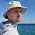### Mixed Models in R - Bigger, Faster, Stronger

When you start doing more advanced sports analytics you'll eventually starting working with what are known as hierarchical, nested or mixed effects models. These are models that contain both fixed and random effects. There are multiple ways of defining fixed vs random random effects, but one way I find particularly useful is that random effects are being "predicted" rather than "estimated", and this in turn involves some "shrinkage" towards the mean.

Here's some R code for NCAA ice hockey power rankings using a nested Poisson model (which can be found in my hockey GitHub repository):
model <- gs ~ year+field+d_div+o_div+game_length+(1|offense)+(1|defense)+(1|game_id)
fit <- glmer(model,
data=g,
verbose=TRUE,
)

The fixed effects are year, field (home/away/neutral), d_div (NCAA division of the defense), o_div (NCAA division of the offense) and game_length (number of overtime periods); offense (strength of offense), defense (strength of defense) and game_id are all random effects. The reason for modeling team offenses and defenses as random vs fixed effects is that I view them as random samples from the same distribution. As mentioned above, this results in statistical shrinkage or "regression to the mean" for these values, which is particularly useful for partially completed seasons.

One of the problems with large mixed models is that they can be very slow to fit. For example, the model above takes several hours on a 12-core workstation, which makes it very difficult to test model changes and tweaks. Is there any way to speed up the fitting process? Certainly! One way is to add two options to the above code:

fit <- glmer(model,
data=g,
verbose=TRUE,
nAGQ=0,
control=glmerControl(optimizer = "nloptwrap")
)

What do these do? Model fitting is an optimization process. Part of that process involves the estimation of particular integrals, which can be very slow; the option "nAGQ=0" tells glmer to ignore estimating those integrals. For some models this has minimal impact on parameter estimates, and this NCAA hockey model is one of those. The second option tells glmer to fit using the "nloptwrap" optimizer (there are several other optimizers available, too), which tends to be faster than the default optimization method.

The impact can be rather startling. With the default options the above model takes about 3 hours to fit. Add these two options, and the model fitting takes 30 seconds with minimal impact on the parameter estimates, or approximately 400 times faster.

1.thanks a lot!! love you, you made my day!

2.I am very new to GLMs, and GLMMs. But, I'm trying to analyze some data we gather in our lab. We have one categorical dependent variable (accuracy in a 2AFC) and three nominal independent variables, treatment condition (4 levels), order of presentation of treatment condition( 4 levels), and distractor type in the 2AFC (3 levels). Each subject (n=24) participated in each of the treatment conditions, and each treatment condition had exactly 3 instances of the each of the levels of the distractor. Order of treatment was fully counterbalanced. So, I fit a glmer model of the form

modela = glmer(accuracy ~ foil + condition + order + foil:condition:order + order:condition + order:foil + condition:order + (1|subnum), data=mydata,REML=FALSE, family="binomial")

but that failed to converge. So, I tried your two options,

modeld = glmer(accuracy ~ order+condition+foil + condition:foil + order:condition + foil:order + condition:foil:order + (condition + foil + order|subnum), data=mydata, family = "binomial", control=glmerControl(optimizer="bobyqa"), nAGQ=0)

and that returns with a warning "maxfun < 10 * length(par)^2 is not recommended."

I can call summary on both models, but the estimates and their z-values end up being quite different. Any idea of what's going wrong here?

3.Thank you very much. This kinda changed everything in my research.

4.### A Bayes' Solution to Monty Hall

For any problem involving conditional probabilities one of your greatest allies is Bayes' Theorem. Bayes' Theorem says that for two events A and B, the probability of A given B is related to the probability of B given A in a specific way.

Standard notation:

probability of A given B is written $$\Pr(A \mid B)$$
probability of B is written $$\Pr(B)$$

Bayes' Theorem:

Using the notation above, Bayes' Theorem can be written: $\Pr(A \mid B) = \frac{\Pr(B \mid A)\times \Pr(A)}{\Pr(B)}$Let's apply Bayes' Theorem to the Monty Hall problem. If you recall, we're told that behind three doors there are two goats and one car, all randomly placed. We initially choose a door, and then Monty, who knows what's behind the doors, always shows us a goat behind one of the remaining doors. He can always do this as there are two goats; if we chose the car initially, Monty picks one of the two doors with a goat behind it at random.

Assume we pick Door 1 and then Monty sho…

### Gambling to Optimize Expected Median Bankroll

Gambling to optimize your expected bankroll mean is extremely risky, as you wager your entire bankroll for any favorable gamble, making ruin almost inevitable. But what if, instead, we gambled not to maximize the expected bankroll mean, but the expected bankroll median?

Let the probability of winning a favorable bet be $$p$$, and the net odds be $$b$$. That is, if we wager $$1$$ unit and win, we get back $$b$$ units (in addition to our wager). Assume our betting strategy is to wager some fraction $$f$$ of our bankroll, hence $$0 \leq f \leq 1$$. By our assumption, our betting strategy is invariant with respect to the actual size of our bankroll, and so if we were to repeat this gamble $$n$$ times with the same $$p$$ and $$b$$, the strategy wouldn't change. It follows we may assume an initial bankroll of size $$1$$.

Let $$q = 1-p$$. Now, after $$n$$  such gambles our bankroll would have a binomial distribution with probability mass function \[ \Pr(k,n,p) = \binom{n}{k} p^k q^{n-k…